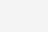# 【深度学习】吴恩达课程笔记(二)——浅层神经网络、深层神经网络

【深度学习】吴恩达课程笔记(二)——浅层神经网络、深层神经网络笔记专栏链接 【深度学习】吴恩达课程笔记(一)——深度学习概论、神经网络基础 四、浅层神经网络 1.双层神经网络表示 x1 ,x2 ,x3：输入层A，指的是单个样本的输入值 中间四个神经元：隐藏

【深度学习】吴恩达课程笔记(一)——深度学习概论、神经网络基础

# 四、浅层神经网络

## 1.双层神经网络表示x1 ,x2 ,x3：输入层A，指的是单个样本的输入值

• 正向传播

• 训练样本分别对隐藏层的各神经元的参数（w向量和b值）进行计算得到z
• 各神经元的z放到一起组成Z
• z激活后得到a
• 各神经元的a放到一起组成A

$z^{}_{1}=w^{T}_{1}x+b^{}_{1},a^{}_{1}=σ(z^{}_{1})\\ z^{}_{2}=w^{T}_{2}x+b^{}_{2},a^{}_{1}=σ(z^{}_{2})\\ z^{}_{3}=w^{T}_{3}x+b^{}_{3},a^{}_{1}=σ(z^{}_{3})\\ z^{}_{4}=w^{T}_{4}x+b^{}_{4},a^{}_{1}=σ(z^{}_{4})\\$• 各神经元的A再作为训练样本对对输出层的单个神经元的参数（w向量和b值）进行计算得到z
• z激活得到a

$Z^{}=W^{}X+b^{}\\ A^{}=σ(Z^{})\\ Z^{}=W^{}A^{}+b^{}\\ A^{}=σ(Z^{})$
• 反向传播

• 从输出结果到第二层到第一层依次计算对成本函数的导数，达到对各个w、b的迭代、训练效果

## 2.双层神经网络的前向传播

n：第n层的单元数，n表示特征向量x的维度### 第二层前向传播

$\textcolor{red}{第一层}\\ X.shape=(n^{},m)\\ W^{}.shape=(n^{},n^{})\\ b^{}.shape=(n^{},1)\\ Z^{}.shape=(n^{},m)\\ A^{}.shape=(n^{},m)\\ \textcolor{red}{第二层} \\ W^{}.shape=(n^{},n^{})\\ Z^{}.shape=(n^{},m)\\ A^{}.shape=(n^{},m)\\ Y.shape=A^{}.shape=(n^{},m)$

## 3.双层神经网络的反向传播

### 参数

$训练样本维数：n^{} \\ 隐藏层神经元个数：n^{} \\ 输出层神经元个数：n^{}=1 \\ W^{}:(n^{},n^{})\\ b^{}:(n^{},1)\\ W^{}:(n^{},n^{})\\ b^{}:(n^{},1)\\ 成本函数：J(W,b)=\frac{1}{m}\sum_{i=1}^{m}{L(ŷ_i,y_i)}$

### 梯度下降

$dW^{[i]}=\frac{\partial J}{\partial W^{[i]}},db^{[i]}=\frac{\partial J}{\partial b^{[i]}}\\ W^{[i]}=W^{[i]}-\alpha dW{[i]} \\ b^{[i]}=b^{[i]}-\alpha db{[i]}\\ i=1,2$

### 反向传播公式

$dZ^{}=A^{}-Y\\ dW^{}=\frac{1}{m}dZ^{}A^{T}\\ db^{}=\frac{1}{m}np.sum(dZ^{},axis=1,keepdims=True)\\ dZ^{}=W^{T}dZ^{}*g^{’}(Z^{})\\ dW^{}=\frac{1}{m}dZ^{}X^{T}\\ db^{}=\frac{1}{m}np.sum(dZ^{},axis=1,keepdims=True)\\$

### 第二层反向传播推导## 4.激活函数

1. sigmoid：只可能用于二元分类的输出层。

$a=\frac{1}{1+e^{-z}}\\ \frac{da}{dz}=a(1-a)$2. tanh：几乎在所有情况下优于sigmoid函数。（计算速度更快）

$a=\frac{e^{z}-e^{-z}}{e^{z}+e^{-z}}\\ \frac{da}{dz}=1-a^2$3. ReLU(Rectified Linear Unit)：最常用的默认激活函数

a=max(0,z)\\ \frac{da}{dz}=\left\{ \begin{aligned} 0 & , z<0 \\ 1 & , z>0 \\ undefined&,z=0 \end{aligned} \right.4. leaky ReLU：有人认为这个比ReLU好

a=max(\alpha z,z),\alpha \ usually \ less\ than\ 1\\ \frac{da}{dz}=\left\{ \begin{aligned} \alpha & , z<0 \\ 1 & , z>0 \\ undefined&,z=0 \end{aligned} \right.## 5.为什么要使用非线性激活函数？

• 解决线性不可分问题：线性激活函数（如恒等映射）只能产生线性变换，无法处理非线性可分的问题。
• 增强模型的表达能力：非线性激活函数能够引入非线性变换，使得神经网络能够学习更加复杂的模式和特征。
• 防止梯度消失：在深层神经网络中，使用线性激活函数会导致梯度逐层地缩小，进而导致梯度消失的问题。
• 增加模型的非线性响应：非线性激活函数可以引入非线性响应，使得模型能够更好地适应数据的非线性特征。这对于处理图像、语音等复杂数据具有重要意义，能够提高模型的性能。

## 6.为什么要对W随机初始化？

• 如果把W初始化为全部为0，那么第一层上的神经元训练后都将是相同的，其下一层的神经元对上一层的判断权重也是完全相同的，同时这一层的神经元也会是完全相同的。由归纳法，每一层上的神经元都是完全相同的。这样就丧失了多层神经网络的判断性能优势。
• 初始化时应该使W中的数字尽量小，以使得sigmoid或tanh计算导数时处于导数较大的区域，以保证迭代学习的速度

# 五、深层神经网络

## 1.变量定义

l 层数
n[l] l 层的单元数## 2.矩阵的维数

X (n,m)
W[l] and dW[l] (n[l],n[l-1])
b[l] and db[l] (n[l],1)
Z[l] and dZ[l] (n[l],m)
A[l] and dA[l] (n[l],m)
Y (n[the last l ],m)

## 3.为什么使用深层表示（Deep Representation）

1. 特征表达能力增强：深层表示可以通过逐层的非线性变换，将原始输入数据转化为更高级别的抽象特征表示。每一层都可以学习到数据的不同抽象层次的特征，使得模型能够更好地捕捉输入数据中的结构和模式。相比于浅层模型，深层表示具有更强大的特征表达能力。
2. 特征的层次化表示：深层表示可以将输入数据的特征表示分解为多个层次，每一层都对应着不同抽象层次的特征。这种层次化的特征表示使得模型能够更好地理解数据的结构和语义，从而提高模型的泛化能力和鲁棒性。
3. 梯度传播更有效：在深层网络中，通过反向传播算法计算梯度时，梯度可以更容易地传播到较早的层。这是因为深层网络中的参数共享和权重共享的结构，使得梯度能够通过多个层级的连接路径传递。相比于浅层网络，深层网络可以更有效地利用梯度信息进行参数更新，从而提高模型的训练效率和性能。
4. 数据表示的可分离性：深层表示可以将输入数据的不同方面进行分离和表示。例如，在图像处理任务中，底层的卷积层可以学习到边缘和纹理等低级特征，而高层的全连接层可以学习到物体的形状和类别等高级特征。这种分离性使得模型能够更好地对不同方面的特征进行建模和学习。

## 4.深层神经网络块图解## 5.深层神经网络前向和反向传播的实现

$A^{}=X\\ Z^{[l]}=W^{}A^{[l-1]}+b^{[l]}\\ A^{[l]}=g^{[l]}(Z^{[l]})\\$

$\textcolor{red}{}\\ dZ^{[l]}=dA^{[l]}*g^{[l]’}(Z^{[l]})\\ dW^{[l]}=\frac{1}{m}dZ^{[l]}A^{[l-1]T}\\ db^{[l]}=\frac{1}{m}np.sum(dZ^{[l]},axis=1,keepdims=True)\\ dA^{[l-1]}=W^{[l]T}dZ^{[l]}$

(0)

### 相关推荐

• #### spring的事务和mysql的事务_spring事务什么时候提交

spring的事务和mysql的事务_spring事务什么时候提交本文分享一些关于Mysql如何解决多事务并发的问题和Spring源码是怎么控制事务以及一些事务失效的场景。 分享内容： Mysql事务隔离机制 锁机制 MVCC多版本并发控制隔离 Spring事务应用

• #### 可能是最漂亮的Spring事务管理详解[亲测有效]

可能是最漂亮的Spring事务管理详解[亲测有效]事务是逻辑上的一组操作，要么都执行，要么都不执行. 原子性： 事务是最小的执行单位，不允许分割。事务的原子性确保动作要么全部完成，要么完全不起作用； 持久性: 一个事务被提交之后。它对数据库中数据的改变是持久的，即使数据库发生故障也不应该对其有任何影响。 所谓事务管理，其实就是…

• #### macbook窗口快捷键_数据库事务并发

macbook窗口快捷键_数据库事务并发talbelock表锁是mysql最基本的锁策略，也是开销最小的锁，它会锁定整个表；具体情况是：若一个用户正在执行写操作，会获取排他的“写锁”，

• #### wireshark中文使用教程（网络抓包工具wireshark）

wireshark中文使用教程（网络抓包工具wireshark）

• #### Matlab实现神经网络

Matlab实现神经网络神经网络是一种模仿人类神经系统，以处理信息为目的的计算模型。它由大量节点（或称神经元）和连接它们的边组成，每个节点代表一个变量，边表示变量之间的关系。在神经网络中，信息通过节点之间的连接传递，并在各个

• #### Python代码笔记——excel格式题库转word格式题库

Python代码笔记——excel格式题库转word格式题库本篇代码片段是由Python加pandas库，python-docx完成，因为近日，对象的单位需要考试，所以发给他们每个人excel格式的题库，

• #### c++选择排序算法代码_java排序代码

c++选择排序算法代码_java排序代码前言:排序就是重新排列表中的元素，是表中的元素满足按关键字递增或递减的过程。为了查找方便，通常要求这些通常要求计算机中的，表示按关键字有序的算法

• #### 雨滴桌面秀下载（雨滴怎么下载）[通俗易懂]

雨滴桌面秀下载（雨滴怎么下载）[通俗易懂]